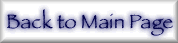## 1. Introduction and Importance of Form Factors

The complex form factor f is the fundamental parameter for all optical devices. It specifies refractive indices, permittivities, scattering and attenuation coefficients, and hence the critical properties for mirrors, lenses, filters and coatings. At higher (x-ray) photon energies, the form factor becomes accessible to theoretical prediction on the basis of atomic physics and the atomic form factor .

In the x-ray energy range covered herein, the primary interactions of photons with atoms are photoabsorption and coherent (elastic) scattering. Inelastic (Compton) scattering becomes dominant for all elements as the higher γ-ray energies are approached. For light elements, this transfer of dominance occurs at much lower energies (for hydrogen the inelastic component dominates above 3 keV to 5 keV). Additional nuclear scattering and absorption occurs above MeV energies, including pair production and Delbrück scattering from the nuclear field; and nuclear resonant processes (such as nuclear Thomson scattering) . For XUV photons below the energy range of this paper, lattice phonon absorption, delocalized plasmon excitation, excitons and dipole resonances may appear . Although these remain qualitatively identifiable as photon interactions with bound electrons, it is misleading to attempt to identify them with atomic orbitals or isolated atoms.

In the intermediate energy range, typically from 0.01 keV to 0.1 keV through to 80 keV to 800 keV, the interaction of the incident photon with the electrons - i.e., with the bound atomic orbitals - without production of secondary x rays of reduced energy, is the dominant process. The photon is then either scattered without altering the internal energy of the atom, or it is fully absorbed. This absorption is usually into a single atomic orbital, with a consequent ejection of a photoelectron and production of a singly-ionized species.

Photoabsorption and (Rayleigh) scattering are both described by the structure factor F of the material in condensed or gas phase. Diffracted intensity or coherent scattering is a complicated function of F, but for weak reflections is linear or quadratic in F. Equally, transmission through a bulk material is a complex function of F but local attenuation is a relatively simple function of the imaginary component of F [4,5].

This is well known in the crystallographic community and is used extensively in the multilayer community at lower energies [6-8]. The structure factor for a given reflection (denoted hkl from the Miller indices) is a sum over the atoms in the appropriate lattice (for a crystal) of the atomic form factors or the x-ray scattering factors fj of the jth atom:(eq 1)

where thermal diffuse scattering [TDS, (eq 17), discussed below] is neglected, Mj is the thermal parameter for the given temperature, reflection and atom, and the location of the atom in the unit cell is given by (xj, yj, zj). For an isolated atom or a single elemental lattice, a scaled atomic form factor may therefore be substituted for the structure factor.

At grazing angles of incidence with solids, photons interact with the surface, and the photoabsorption and reflection processes may be given by Fresnel equations (while still dominated by electron orbital interaction and governed by the structure factor and form factors) .

If the atoms in a condensed system may be considered to scatter as dipoles (i.e., for low energies or small scattering angles) then the interaction of x rays with matter may be described using optical constants such as the complex index of refraction nr or the complex dielectric constant ε(E), which are related to the form factors by(eq 2)

where nj is the atom number density and re is the classical electron radius.